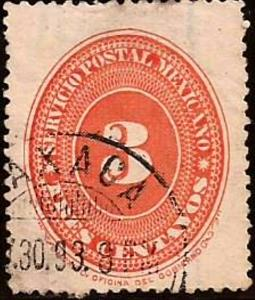## Image Colnect-2788-333-Numeral-of-value.jpgFull resolution (744 x 875 pixels) Type: stamp Issuer: Mexico Date: 1892 Denom: ? Visibility: public Image owner: none 10 matches: postage 3c org issue=1892a d=numeral (1886) wmk=CORREOS E U M paper=blue lines postage 4c org issue=1892a d=numeral (1886) wmk=CORREOS E U M paper=blue lines postage 6c org issue=1892a d=numeral (1886) wmk=CORREOS E U M paper=blue lines postage 10c org issue=1892a d=numeral (1886) wmk=CORREOS E U M paper=blue lines postage 20c org issue=1892a d=numeral (1886) wmk=CORREOS E U M paper=blue lines postage 25c org issue=1892a d=numeral (1886) wmk=CORREOS E U M paper=blue lines postage 5p blgrn issue=1892b d=Hidalgo profile wmk=CORREOS E U M paper=blue lines postage 5p car issue=1892b d=numeral (1892 Mexico) wmk=CORREOS E U M paper=blue lines postage 10p blgrn issue=1892b d=Hidalgo profile wmk=CORREOS E U M paper=blue lines postage 10p car issue=1892b d=numeral (1892 Mexico) wmk=CORREOS E U M paper=blue lines Object 40063 ( postage 3c org issue=1892a d=numeral (1886) wmk=CORREOS E U M paper=blue lines) Object 40064 ( postage 4c org issue=1892a d=numeral (1886) wmk=CORREOS E U M paper=blue lines) Object 40065 ( postage 6c org issue=1892a d=numeral (1886) wmk=CORREOS E U M paper=blue lines) Object 40066 ( postage 10c org issue=1892a d=numeral (1886) wmk=CORREOS E U M paper=blue lines) Object 40067 ( postage 20c org issue=1892a d=numeral (1886) wmk=CORREOS E U M paper=blue lines) Object 40068 ( postage 25c org issue=1892a d=numeral (1886) wmk=CORREOS E U M paper=blue lines) Crops No visible images cropped from this one. Measurements Resolution is unknown. Source Source name: Colnect File URL: http://i.colnect.net/b/2788/333/Numeral-of-value.jpg Uploaded November 15, 2015, 3:07 pm by Stan Shebs